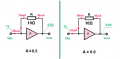# Bootstrap capacitor increase input impedance of voltage follower ...how??

#### Himanshoo

Joined Apr 3, 2015
265
Hello guys ...

According to theory in order to increase the input impedance bootstrap capacitor is employed…which couples the same output voltage to point B ..and as the circuit is a voltage follower Vin = Vout ..thus the voltage difference across R1 is very small ..which means that no or very little current will flow through R1 ..which can be also visualised as a very high resistance present..

Analysis:

Without bootstrap capacitor Cb:

Zin = R1; figure 1..

With bootstrap capacitor:

Zin = (1+Aol)R1 ....where Aol = open loop gain of opamp.

As its clear from above equation that input impedance increase by a factor of (1+Aol)..

since from figure 1. we have

i1 = V1/R1 = 500mv / 68K = 7.35 mA.

from figure 2 ..we have

Zin = (999.996 - 999.998)mV / 7.35mA (forget Zin = (1+Aol) R1 for the time being)
= 2.72* 10^-4 k

But the value of Zin should be very 2 large ..but is not agreeing with the theory.. why?? please justify..

Last edited:

#### Alec_t

Joined Sep 17, 2013
13,060
from figure 2 ..we have Zin = (999.996 - 999.998)mV / 7.35k
How do you arrive at that?

#### Himanshoo

Joined Apr 3, 2015
265
How do you arrive at that?
the way iam thinking is that ...no matter how much resistance is connected in series... current i1(in this case wont change )
as it is very usual to think that Cb is making R1 look like a very high resistance as the voltage difference across R1 is very small i.e(999.996-999.998) ..its ok uptil now as a very small current would flow across R1..

since current i1 in both the cases are same..and the voltage difference is very low....hence it force me to think that now Zin might have increased..as Zin is literally equal to R1 (in absence of Cb)..simple ohm law..

#### Jony130

Joined Feb 17, 2009
5,405
Your equation is wrong. Please notice that for frequency larger than R1||R2 Cb act just like a short circuit.
So R1 resistor is connected between the input and the output of the amplifier. The voltage across R1 resistor is equal to the difference between Vin and Vout.
So the situation looks like thisNow let as try to find a input resistance.

Rin = Vin/Iin

In = (Vin - Vout)/R = (Vin - A*Vin)/R = Vin * (1 - A)/R

Rin = Vin/Iin = R/(1 - A)

So if Vin = 1V and A = 0.5V/V we get 0.5V at the output. So the input current is equal to:

Iin = (Vin - Vout)/R = 0.5V/10Ω = 50mA and therefore

Rin = 1V/50mA = 20Ω

If we increase the gain to 0.9V/V we have

Iin = (Vin - Vout)/R = 0.1V/10Ω = 10mA

So the input resistance is equal to

Rin = 1V/10mA = 100Ω

•Himanshoo

#### Himanshoo

Joined Apr 3, 2015
265
I spot it ...what i misunderstood was that Zin depends upon (Vin-Vout)..which isn't the situation actually it depends on Vin...hence you are right Jony ...instead Iin depends on R1

frequency larger than R1||R2 Cb act just like a short circuit.
what does this mean...are you talking in terms of impedance...please clarify...

#### Jony130

Joined Feb 17, 2009
5,405
I spot it ...what i misunderstood was that Zin depends upon (Vin-Vout)..which isn't the situation actually it depends on Vin...hence you are right Jony ...instead Iin depends on R1
Also notice that in fact Rin is equal to (R1/(1 - A))||Rint where Rint is a amplifier input resistance. Because only if Rint = ∞-->Rin = R1/(1 - A)

Also what will happens if the amplifier gain is negative (inverting amplifier)?

what does this mean...are you talking in terms of impedance...please clarify...
I was trying to say that for a frequency where Xcb << R1||R2 - the Rin is equal to R1/(1 - A). But for the lower frequency Rin = R1+R2.

•Himanshoo

#### Himanshoo

Joined Apr 3, 2015
265

#### Himanshoo

Joined Apr 3, 2015
265
Also what will happens if the amplifier gain is negative (inverting amplifier)?
The input impedance in that case would be ...Zin=[1+(Aol/Acl)]R1...since i am not completely sure of it...as i refer book named as"Operational Amplifier and Linear ICs"..by david bell...I dont know that the book requires some serious proof reading.....

Instead I urge you take a look over it....coz I fear that I am learning some kind of crap...

#### Jony130

Joined Feb 17, 2009
5,405
which one?
This one
Zin = (1+Aol)R1

The input impedance in that case would be ...Zin=[1+(Aol/Acl)]R1...since i am not completely sure of it...as i refer book named as"Operational Amplifier and Linear ICs"..by david bell...I dont know that the book requires some serious proof reading.....
Instead I urge you take a look over it....coz I fear that I am learning some kind of crap...
This time your equation ([1+(Aol/Acl)]R) only work for voltage-series (series shunt) feedback amplifiers.
And this has only a effect on a resistor inside a feedback loop (only on amplifier input resistance).
•